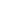Free Trial   |Purchase   |  Products   |  Downloads   |  Support# Indexing on Numerical Fields

A common problem in working with indexes is that people attempt to index on numeric fields types without taking the extra step of using the STR( <field>, <width>) function to pad the data. FoxPro supports calling STR( <field> ) without specifying a width, however Apollo requires a width to be specified.

The following works fine under FoxPro:
INDEX = STRINGFLD1 + STR(NUMERICFLD) + STRINGFLD2

The equivalent under Apollo is:
INDEX = STRINGFLD1 + STR(NUMERICFLD, 10) + STRINGFLD2

The value of NUMERICFLD could be different sizes. So, if we assume both STRINGFLD fields are CHAR(10), we would get the following:

Rec 1:
STRINGFLD1 = "Charlie"
NUMERICFLD = 268323
STRINGFLD2 = "Boo"

Rec 2:
STRINGFLD1 = "Bob"
NUMERICFLD= 57
STRINGFLD2="Pooky"

Using the above index expression, the index values would equate to (# is space):

"Charlie###268323Boo#######
"Bob#######57Pooky#####

These index results are different sizes - the second is 2 bytes shorter which results in bad index values leading to incorrect search results. However if you use the STR( <field>, <width>) as STR( NUMERICFLD, 10), you would get the expected result:

"Charlie###268323####Boo#######"
"Bob#######57########Pooky#####"

Both index results are the same size. The same technique applies to the PADL(), PADR(), PADC() padding functions: you would need to make the numeric expression a consistent string width. You need to apply the same logic to your search and scope values and make the values being searched conform to your index expression.

### Summary

mynumfield
age
sales

Index numeric fields as follows:
STR( mynumfield, 6)
STR( age, 3)
STR( sales, 10)

You can also combine numeric fields with non-numeric fields as follows:
STR( mynumfield, 6)
LNAME + STR( age, 3)
STATE + STR( sales, 10) + SALESREPProducts Technologies Support Company